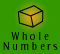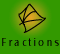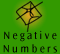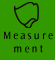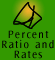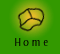| Measuring volume and capacity | Volume and capacity measurement facts |
| Summary of volume formulae of some shapes | Quick quiz |

Measuring volume and capacity

Volume is the amount of space an object occupies whereas capacity is the amount of a fluid that an object can hold, i.e., capacity measures the "internal volume" of a container. A jug has both volume and capacity since it takes up space and can also hold an amount of liquid. A block of wood has volume but no capacity because it takes up space but cannot hold anything. These terms are often confused.

There are two sets of (connected) units. Litres are measured for volume of liquids and for the capacity of containers. Cubic metres, cubic centimetres etc are used for volumes of objects, spaces, etc.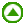Volume and capacity measurement facts

VOLUME SI unit: cubic metre (m3)
1000 cubic centimetres (cm3) = 1 cubic decimetre (dm3)

= 1 litre (L)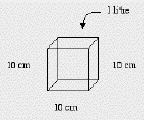1 cm3 = 1 L
1000 cubic decimetres (dm3)

= 1 cubic metre (m3)

= 1 kilolitre (kL)

Alternatively, especially for gases and liquids:

 1 cubic centimetre (cm3) = 1 millilitre (mL) 1000 millilitres (mL) = 1 litre (L) 1000 litres (L) = 1 kilolitre (kL) = 1 cubic metre (m3) 1000 kilolitres (kL) = 1 megalitre (ML)Summary of volume formulae of some shapes:

 Volume of a cube = L x L x L = L3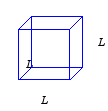To find the volume of a cube we need to multiply together its 3 dimensions of length, width and height. As they are the same for a cube, we can simplify the formula to, the volume of a cube is its length cubed.

Finding the volume of a prism

What is a prism?

To understand how to find the volume of a prism we first need to understand what a prism is!

 These solids are prisms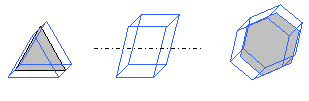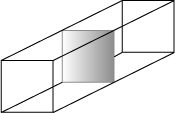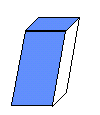Prisms are special solids. They have two polygonal bases joined by parallelograms. The polygonal bases give the uniform cross-sections. This means that if we cut a prism through parallel to the base, each cut piece will be exactly the same size and shape. Can you see why all these solids are prisms?

Example 1: Is this solid a prism?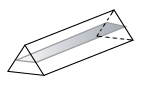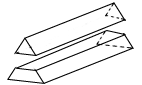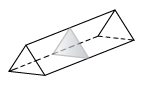Figure 1 Figure 2 Figure 3

 Thinking To find out if this solid is a prism, we need to imagine cutting the solid through parallel to a face to see if the faces of the cut will be exactly the same size and shape if I take several cuts parallel to that face. Looking at figures 1 and 2, we can see that if the solid is cut several times parallel to the face it is sitting on, the resulting cut faces will all be rectangular but they will be different sizes. However, looking at figure 3, we can see that cuts parallel to the end faces will always result in cut faces which are exactly the same size and shape. Therefore this solid is a prism.

 These solids are not prisms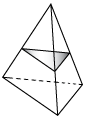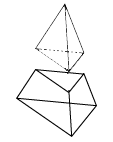If these solids are cut crossways several times parallel to the face they are sitting on, the cut faces will be different. There is no plane through which the solids can be cut to produce uniform cross-sections.

 Volume of a prism = Area x height We can find the volume of a prism by multiplying the area of the uniform cross section by the height, where the height is measured perpendicular to the uniform cross section. It is because the cross-section is uniform across the whole shape that we can multiply the area of the cross-section by the height of the shape.

 Volume of a rectangular prism = L x W x H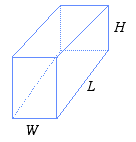We could also find the volume of a rectangular prism using the formula for prisms, A x h. Can you see why?

Although by our definition a cylinder is not a prism, we can use the same formula to calculate the volume of a cylinder as we have used for a prism because a cylinder also has a uniform cross-section.

Example 2: Justification for the formula for the volume of a cylinder

The solids pictured below are not prisms, but we can see that they do have a uniform cross-section. This means that if we know the area of the base we can use the same formula to calculate the volume, V = Area of cross-section x h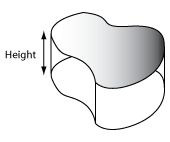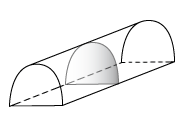Volume of a pyramid = 1/3 x A x h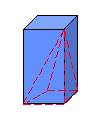The volume of a pyramid is 1/3 of the volume of an enclosing prism, i.e. the volume of the red pyramid 1/3 of the volume of the blue prism, so 1/3 of the area of the base multiplied by its height. This remarkable fact also applies to cones: the volume of a cone is one third of the volume of the enclosing cylinder.

Example 3: Find the volume of a pyramid which has a triangular base area of 5.3 cm2 and a height of 9 cm.

 Setting Out Thinking V = 1/3 x A x h V = 1/3 x 5.3 x 9 V = 15.9 cm3 I know the area of the base of the pyramid is 5.3 cm2. The formula for the volume of a pyramid is 1/3 x area of the base x height. Therefore the volume is 1/3 x 5.3 x 9 = 15.9 cm3

 Volume of a cylinder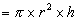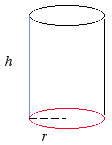We know that the area of a circle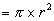and therefore to find the volume of a cylinder we just need to multiply the area of the circle by the height of the cylinder. (Knowing that a cylinder is a solid of uniform cross-section also means that we know that its volume is the area of its base x height.)

Example 4: Find the capacity of a cylindrical water tank which has a radius of 0.75 m and a height of 2.3 m.

 Model Setting Out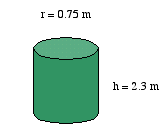Volume of a cylinder= 3.1416 x 0.75 x 0.75 x 2.3 = 5.43 m3 ( I have rounded my answer to 2 decimal places) The units of our answer are cubic metres, but as we are interested in the capacity of the tank it makes more sense to think of how many litres will fit into the tank. We can convert our answer from cubic metres to litres. 1 cubic metre = 1000 litres = 1 kilolitre So, 5.43 cubic metres = 5.43 x 1000 litres = 5430 litres.

Volume of a cone =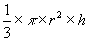Example 5: Calculation of the volume of a cone and conversion of units.
Yuping loves ice-cream! She wants to find out if she will get more ice-cream in a cone compared to a small bucket. She has purchased both to perform the test. Yuping measured the dimensions of her ice-cream cone and found that it was 12 cm in height and had a radius of 4.5 cm.
If the ice-cream was densely packed into the cone, how much ice-cream would the cone hold?
If the bucket holds 150 ml of ice-cream, which holds more?

Setting Out
 volume of a cone == 1/3 x 3.142 x (4.5 cm)2 x 9 cm = 190.8765 cm3
Thinking
We have found that the cone holds 190.8765 cm3 of ice-cream. This is assuming that the ice-cream is very well packed into the cone. We know that the bucket holds 150 ml of ice-cream. To compare the two objects we must do so using the same units. We know that 1 cm3 = 1 ml, so the cone can hold approximately 190 ml of ice-cream.

So if the cone can hold 190 ml of ice-cream and the bucket holds 150 ml of ice-cream which holds more? Theoretically the cone holds more ice-cream but it is unlikely that the ice-cream would be so tightly packed as to occupy the entire volume of the cone. On the other hand you would expect an extra scoop of ice-cream on top of the cone!

 Volume of a sphere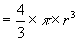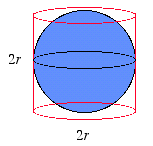A neat relationship between the volume of a sphere and a cylinder. Recall that the volume of a cylinder =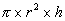If the height of the cylinder is equal to twice the radius then the formula for the volume can be simplified to volume =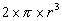The volume of a sphere is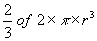So the volume of the sphere is 2/3 of the volume of the enclosing cylinder. You can use sand and water to show this with plastic models.Quick quiz

 1. What is the volume of each of these solids? r = 3 m h = 10 cm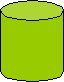h = 7.5 m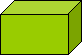A = 6 cm2 2. If the perpendicular height of the rectangular prism, pictured below, is 8 m and the side length of its square base is 4 m, what is its volume?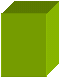3. If we know that the area of the hexagonal faces of this solid is 9 cm2 and it is 5 cm thick, what is its volume?